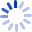# Linear Probability Logit & Probit Models

Ordinary regression analysis is not appropriate for investigating dichotomous or otherwise "limited" dependent variables, but this volume examines three techniques -- linear probability, probit, and logit models -- which are well-suited for such data. It reviews the linear probability model and discusses alternative specifications of non-linear models. Using detailed examples, Aldrich and Nelson point out the differences among linear, logit, and probit models, and explain the assumptions associated with each.

##### Cash for Textbooks